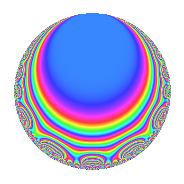# Properties

 Label 122.2.aLevel 122 Weight 2 Character orbit a Rep. character $$\chi_{122}(1,\cdot)$$ Character field $$\Q$$ Dimension 6 Newforms 3 Sturm bound 31 Trace bound 1

# Related objects

## Defining parameters

 Level: $$N$$ = $$122 = 2 \cdot 61$$ Weight: $$k$$ = $$2$$ Character orbit: $$[\chi]$$ = 122.a (trivial) Character field: $$\Q$$ Newforms: $$3$$ Sturm bound: $$31$$ Trace bound: $$1$$ Distinguishing $$T_p$$: $$3$$

## Dimensions

The following table gives the dimensions of various subspaces of $$M_{2}(\Gamma_0(122))$$.

Total New Old
Modular forms 17 6 11
Cusp forms 14 6 8
Eisenstein series 3 0 3

The following table gives the dimensions of the cuspidal new subspaces with specified eigenvalues for the Atkin-Lehner operators and the Fricke involution.

$$2$$$$61$$FrickeDim.
$$+$$$$+$$$$+$$$$1$$
$$+$$$$-$$$$-$$$$2$$
$$-$$$$+$$$$-$$$$3$$
Plus space$$+$$$$1$$
Minus space$$-$$$$5$$

## Trace form

 $$6q$$ $$\mathstrut -\mathstrut 2q^{3}$$ $$\mathstrut +\mathstrut 6q^{4}$$ $$\mathstrut +\mathstrut 2q^{5}$$ $$\mathstrut +\mathstrut 4q^{7}$$ $$\mathstrut +\mathstrut 4q^{9}$$ $$\mathstrut +\mathstrut O(q^{10})$$ $$6q$$ $$\mathstrut -\mathstrut 2q^{3}$$ $$\mathstrut +\mathstrut 6q^{4}$$ $$\mathstrut +\mathstrut 2q^{5}$$ $$\mathstrut +\mathstrut 4q^{7}$$ $$\mathstrut +\mathstrut 4q^{9}$$ $$\mathstrut -\mathstrut 8q^{11}$$ $$\mathstrut -\mathstrut 2q^{12}$$ $$\mathstrut +\mathstrut 2q^{13}$$ $$\mathstrut +\mathstrut 4q^{14}$$ $$\mathstrut -\mathstrut 16q^{15}$$ $$\mathstrut +\mathstrut 6q^{16}$$ $$\mathstrut -\mathstrut 8q^{17}$$ $$\mathstrut -\mathstrut 2q^{19}$$ $$\mathstrut +\mathstrut 2q^{20}$$ $$\mathstrut -\mathstrut 6q^{22}$$ $$\mathstrut +\mathstrut 4q^{23}$$ $$\mathstrut -\mathstrut 4q^{25}$$ $$\mathstrut -\mathstrut 4q^{26}$$ $$\mathstrut -\mathstrut 8q^{27}$$ $$\mathstrut +\mathstrut 4q^{28}$$ $$\mathstrut -\mathstrut 4q^{29}$$ $$\mathstrut -\mathstrut 12q^{30}$$ $$\mathstrut -\mathstrut 4q^{31}$$ $$\mathstrut +\mathstrut 4q^{33}$$ $$\mathstrut -\mathstrut 4q^{34}$$ $$\mathstrut -\mathstrut 12q^{35}$$ $$\mathstrut +\mathstrut 4q^{36}$$ $$\mathstrut -\mathstrut 8q^{37}$$ $$\mathstrut -\mathstrut 4q^{38}$$ $$\mathstrut +\mathstrut 4q^{39}$$ $$\mathstrut -\mathstrut 8q^{41}$$ $$\mathstrut -\mathstrut 12q^{42}$$ $$\mathstrut +\mathstrut 20q^{43}$$ $$\mathstrut -\mathstrut 8q^{44}$$ $$\mathstrut +\mathstrut 18q^{45}$$ $$\mathstrut +\mathstrut 12q^{47}$$ $$\mathstrut -\mathstrut 2q^{48}$$ $$\mathstrut +\mathstrut 38q^{49}$$ $$\mathstrut +\mathstrut 24q^{50}$$ $$\mathstrut +\mathstrut 8q^{51}$$ $$\mathstrut +\mathstrut 2q^{52}$$ $$\mathstrut +\mathstrut 8q^{53}$$ $$\mathstrut -\mathstrut 24q^{54}$$ $$\mathstrut -\mathstrut 8q^{55}$$ $$\mathstrut +\mathstrut 4q^{56}$$ $$\mathstrut +\mathstrut 24q^{57}$$ $$\mathstrut +\mathstrut 6q^{58}$$ $$\mathstrut -\mathstrut 32q^{59}$$ $$\mathstrut -\mathstrut 16q^{60}$$ $$\mathstrut -\mathstrut 2q^{61}$$ $$\mathstrut -\mathstrut 2q^{62}$$ $$\mathstrut +\mathstrut 16q^{63}$$ $$\mathstrut +\mathstrut 6q^{64}$$ $$\mathstrut -\mathstrut 6q^{65}$$ $$\mathstrut +\mathstrut 16q^{66}$$ $$\mathstrut +\mathstrut 28q^{67}$$ $$\mathstrut -\mathstrut 8q^{68}$$ $$\mathstrut -\mathstrut 44q^{69}$$ $$\mathstrut -\mathstrut 2q^{70}$$ $$\mathstrut +\mathstrut 20q^{71}$$ $$\mathstrut +\mathstrut 20q^{73}$$ $$\mathstrut +\mathstrut 22q^{74}$$ $$\mathstrut -\mathstrut 2q^{75}$$ $$\mathstrut -\mathstrut 2q^{76}$$ $$\mathstrut +\mathstrut 6q^{77}$$ $$\mathstrut +\mathstrut 12q^{78}$$ $$\mathstrut -\mathstrut 8q^{79}$$ $$\mathstrut +\mathstrut 2q^{80}$$ $$\mathstrut -\mathstrut 18q^{81}$$ $$\mathstrut +\mathstrut 16q^{82}$$ $$\mathstrut -\mathstrut 14q^{83}$$ $$\mathstrut +\mathstrut 20q^{85}$$ $$\mathstrut +\mathstrut 4q^{86}$$ $$\mathstrut -\mathstrut 6q^{88}$$ $$\mathstrut -\mathstrut 12q^{89}$$ $$\mathstrut +\mathstrut 16q^{90}$$ $$\mathstrut +\mathstrut 24q^{91}$$ $$\mathstrut +\mathstrut 4q^{92}$$ $$\mathstrut +\mathstrut 20q^{93}$$ $$\mathstrut -\mathstrut 28q^{94}$$ $$\mathstrut -\mathstrut 12q^{95}$$ $$\mathstrut +\mathstrut 14q^{97}$$ $$\mathstrut -\mathstrut 8q^{98}$$ $$\mathstrut -\mathstrut 40q^{99}$$ $$\mathstrut +\mathstrut O(q^{100})$$

## Decomposition of $$S_{2}^{\mathrm{new}}(\Gamma_0(122))$$ into irreducible Hecke orbits

Label Dim. $$A$$ Field CM Traces A-L signs $q$-expansion
$$a_2$$ $$a_3$$ $$a_5$$ $$a_7$$ 2 61
122.2.a.a $$1$$ $$0.974$$ $$\Q$$ None $$-1$$ $$-2$$ $$1$$ $$-5$$ $$+$$ $$+$$ $$q-q^{2}-2q^{3}+q^{4}+q^{5}+2q^{6}-5q^{7}+\cdots$$
122.2.a.b $$2$$ $$0.974$$ $$\Q(\sqrt{13})$$ None $$-2$$ $$1$$ $$0$$ $$5$$ $$+$$ $$-$$ $$q-q^{2}+(1-\beta )q^{3}+q^{4}+(-1+\beta )q^{6}+\cdots$$
122.2.a.c $$3$$ $$0.974$$ 3.3.229.1 None $$3$$ $$-1$$ $$1$$ $$4$$ $$-$$ $$+$$ $$q+q^{2}+\beta _{2}q^{3}+q^{4}+(-\beta _{1}-\beta _{2})q^{5}+\cdots$$

## Decomposition of $$S_{2}^{\mathrm{old}}(\Gamma_0(122))$$ into lower level spaces

$$S_{2}^{\mathrm{old}}(\Gamma_0(122)) \cong$$ $$S_{2}^{\mathrm{new}}(\Gamma_0(61))$$$$^{\oplus 2}$$